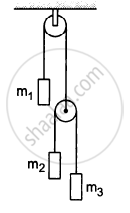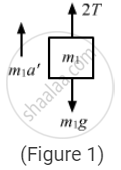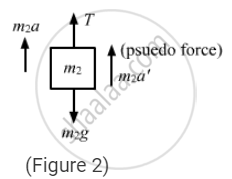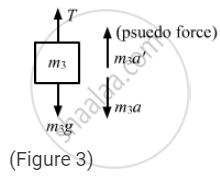# Let M1 = 1 Kg, M2 = 2 Kg and M3 = 3 Kg in Figure (5−E12). Find the Accelerations of M1, M2 and M3. the String from the Upper Pulley to M1 is 20 Cm When the System I - Physics

Sum

Let m1 = 1 kg, m2 = 2 kg and m3 = 3 kg in the following figure. Find the accelerations of m1, m2 and m3. The string from the upper pulley to m1 is 20 cm when the system is released from rest. How long will it take before m1 strikes the pulley?#### Solution

The free-body diagram for mass m1 is shown below:a
The free-body diagram for mass m2 is shown below:The free-body diagram for mass m3 is shown below:Suppose the block m1 moves upward with acceleration a1 and the blocks m2 and m3have relative acceleration a2 due to the difference of weight between them.
So, the actual acceleration of the blocks m1, m2 and m3 will be a1, (a1 − a2) and (a1 + a2), as shown.
From figure 2, T − 1g − 1a1 = 0    ...(i)
From figure 3,$\frac{T}{2} - 2g - 2\left( a_1 - a_2 \right) = 0 . . . \left( ii \right)$
From figure 4,$\frac{T}{2} - 3g - 3\left( a_1 + a_2 \right) = 0 . . . \left( iii \right)$

From equations (i) and (ii), eliminating T, we get:
1g + 1a2 = 4g + 4 (a1 + a2)
5a2 − 4a1 = 3g    ...(iv)

From equations (ii) and (iii), we get:
2g + 2(a1 − a2) = 3g − 3 (a1 − a2)
5a1 + a2 = g    ...(v)

Solving equations (iv) and (v), we get:

$a_1 = \frac{2g}{29}$

$\text{ and }a_2 = g - 5 a_1$

$\Rightarrow a_2 = g - \frac{10g}{29} = \frac{19g}{29}$

$\text{So}, a_1 - a_2 = \frac{2g}{29} - \frac{19g}{29} = - \frac{17g}{29}$

So, accelerations of m1, m2 and m3 are $\frac{19g}{29}\left( up \right), \frac{17g}{29} \left(\text{ down }\right)\text{ and }\frac{21g}{29}\left(\text{ down }\right)$
Now, u = 0, s = 20 cm = 0.2 m
$a_2 = \frac{19g}{29}$
$\therefore s = ut + \frac{1}{2}a t^2$
$\Rightarrow 0 . 2 = \frac{1}{2} \times \frac{19}{29}g t^2$
$\Rightarrow t = 0 . 25 s$

Concept: Newton’s Second Law of Motion
Is there an error in this question or solution?

#### APPEARS IN

HC Verma Class 11, Class 12 Concepts of Physics Vol. 1
Chapter 5 Newton's Laws of Motion
Q 28 | Page 81